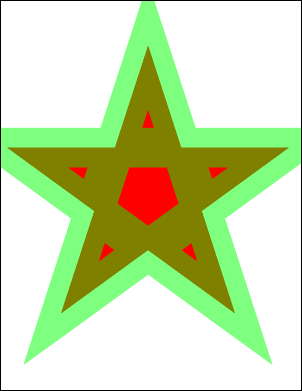AddPoly FunctionAdds a polygon to the current page.Syntax

 [C#] ```int AddPoly(string points, bool filled) int AddPoly(double[] points, bool filled) int AddPoly(double[] points, int index, int count, bool filled)``` [Visual Basic] ```Function AddPoly(points As String, filled As Boolean) As Integer Function AddPoly(points() As Double, filled As Boolean) As Integer Function AddPoly(points() As Double, index As Integer, count As Integer, filled As Boolean) As Integer```Params

 Name Description points The coordinates of the vertices of the polygon. index The index of the first coordinate into the array points. count The number of coordinates in the array points to use. filled Whether to fill the polygon rather than simply outline it. return The Object ID of the newly added Graphic Object.Notes

 Adds a polygon to the current page. The polygon is drawn in the current color at the current width and with the current options. The polygon may be outlined or filled. The points string is a sequence of numbers representing the coordinates of the polygon. The string should be of the format "x1 y1 x2 y2 ... xN yN". The numbers may be delimited with spaces, commas or semicolons. If the first point is equal to the last then the path is closed before outlining. The AddPoly function returns the Object ID of the newly added Graphic Object.Example

 The following code adds a transparent green outlined star over the top of a red filled star.   ```using (Doc doc = new Doc()) {   doc.Width = 80;   doc.Color.String = "255 0 0";   doc.AddPoly("124 158 300 700 476 158 15 493 585 493 124 158", true);   doc.Color.String = "0 255 0 a128";   doc.AddPoly("124 158 300 700 476 158 15 493 585 493 124 158", false);   doc.Save(Server.MapPath("docaddpoly.pdf")); } ``` ```Using doc As New Doc()   doc.Width = 80   doc.Color.String = "255 0 0"   doc.AddPoly("124 158 300 700 476 158 15 493 585 493 124 158", True)   doc.Color.String = "0 255 0 a128"   doc.AddPoly("124 158 300 700 476 158 15 493 585 493 124 158", False)   doc.Save(Server.MapPath("docaddpoly.pdf")) End Using ```docaddpoly.pdf